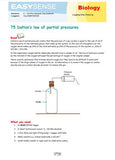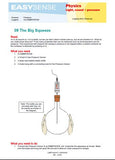# Gas Pressure - Differential 200kPa

datalogging sensors

3141

##### Product Description

The Smart Q Differential Gas Pressure Sensors measure the difference in pressure between two ports.

37-second Gas pressure sensor:  Boyle's law

Normally one port would be a reference and the other connected to the pressure system being monitored.Teaching applications:
• Gay – Lussac’s law (PT)
• Boyle’s law
• Rate of gas production e.g. oxygen from the decomposition of hydrogen peroxide
• Pressure changes in bursting balloon
• Grip test

### Contents/Details:

±200 kPa (Resolution 0.1 kPa)
±30 psi (Resolution 0.02 psi)

If both ports are left open to the atmosphere, the effect of atmospheric pressure will equal each other out and the difference between the ports is zero. If only one port is used, and the unused port is left open to the atmosphere, the Sensor measures pressure above or below atmospheric pressure i.e. gauge pressure. Sample rate up to 20 µS intersample time External diameters of the ports are tapered from 4.0 to 4.3 mm. Use with flexible tubing 3.0 to 3.5 mm internal diameter. These Sensors are not suitable for use with flammable gases.Partial pressures - an experimental approach (Biology (14-18) eBook)
Partial pressures can cause problems for many students. This investigation gives a simple demonstration of the principles of partial pressures.Estimation of absolute zero (Chemistry (14-18) eBook)
The ideal gas laws tell us that if we keep reducing the temperature of a gas then there will come point when the gas occupies no volume and has no pressure. Uses a constant volume, pressure sensor and temperature sensor.

Daltons partial pressure (Chemistry (14-18) eBook)
Partial pressures can cause problems with many students. This investigation gives a simple demonstration of the principles of partial pressures.Uses combustion and removal of resultant CO2.

Molar mass of volatile liquid (Chemistry (14-18) eBook)
The ideal gas law allows us to calculate the molar mass of a gas if we know the volume, pressure and mass. In this investigation acetone is used as it can be made to pass from a liquid to a gas phase at near to room temperatures.

Determination of "R", the gas constant (Chemistry (14-18) eBook)
In this investigation the gas constant R will be calculated. The calculation uses values of pressure from a known volume and mass of carbon dioxide. As the mass of CO2 is known the number of moles can be calculated.

Determination of N for a chemical reaction (Chemistry (14-18) eBook)
The experiment provides the data for students to calculate n for a reaction. This is an application of the gas laws pV = nRT.The big squeeze (pressure in sealed vessel) (Physics (14-18) : Light, Sound & Pressure eBook)
This is a nice, simple, experiment that teaches about the relationship between volume and pressure in a fun way. It can be easily extended to make a teaching experiment about controlling variables in an experiment to produce fair data

An estimation of absolute zero using pressure and temperature changes of a gas (Physics (14-18) : Light, Sound & Pressure eBook)
The investigation attempts to find this point by measuring the pressure of a gas at different temperatures in a fixed volume device. Plotting temperature vs. pressure will allow us to extrapolate the data line back to intercept the temperature axis, The temperature at which the gas occupies no volume is absolute zero.

Daltons partial pressures (Physics (14-18) : Light, Sound & Pressure eBook)
A simple demonstration of partial pressure.

Determination of R (the gas constant) (Physics (14-18) : Light, Sound & Pressure eBook)
In this investigation the gas constant R will be calculated. The calculation uses values of pressure from a known volume and mass of carbon dioxide, from which number of moles present can be determined. Can give a good approximation.

HK\$ 913.00

3141